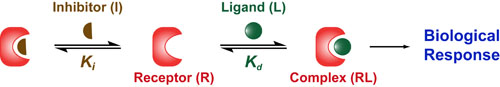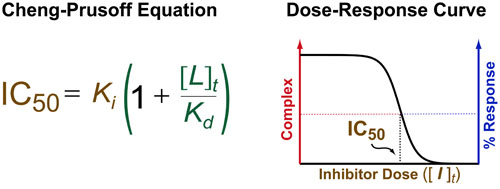# Binding #3: Competitive InhibitionWhile many drugs act like agonists and “turn on” their target/receptor (see posts on Hill Equation and Agonist Limits), many more drugs act like antagonists and “turn off” their target/receptor. One of the most common mechanisms by which drugs “turn off” a target receptor (R) is by blocking the binding of its natural ligand (L). This mechanism is called competitive inhibition and the antagonist is known as an inhibitor (I). Here there are two dissociation constants:the Kd which describes the strength of the interaction between R and L and the Ki which describes the strength of the interaction between R and I.Because inhibitors turn off their target, increasing doses of I reduce the biological activity and the resulting dose-response curves look like the reflection of agonist curves. These curves can be phenomenologically defined by the equation % Inhibition = IC50/ ([I]t + IC50) where the IC50 defines the dose/concentration of inhibitor ([I]t) that elicits 50% inhibition. As a result, the potency of inhibitor-based drugs is described by the IC50 which is mathematically defined by the Cheng-Prusoff Equation.

Unfortunately, like the Hill Equation, the Cheng-Prusoff Equation only works when the receptor concentration is much lower than the Kd and the Ki (i.e. [R]t << Kd,Ki). While this is true in most in vivo and cell-based experiments its sometimes breaks down in many biophysical experiment that require large amounts of protein. Exact formulations of the Cheng-Prusoff Equation and competitive binding equilibria do exist (see references 2-3 below) and will be the subject of future posts..

### REFERENCES:

1. Cheng, Y.; Prusoff, W.H. Relationship between the inhibition constant (KI) and the concentration of inhibitor which causes 50% inhibition (IC50) of an enzymatic reaction. Biochem. Pharmacol. 1973, 22, 3099-3108.
2. Horovitz, A. An accurate method for determination of receptor-ligand and enzyme-inhibitor dissociation constants from displacement curves. PNAS, 1987, 84, 6654-6658.
3. Wang, Z. An exact mathematical expression for describing competitive binding of two different ligands to a protein molecule. FEBS Letters, 1995, 360, 111-114.
4. Segel, I.H. Enzyme Kinetics: Behavior and Analysis of Rapid Equilibrium and Steady-State Enzyme Systems, Wiley-Interscience, 1993